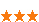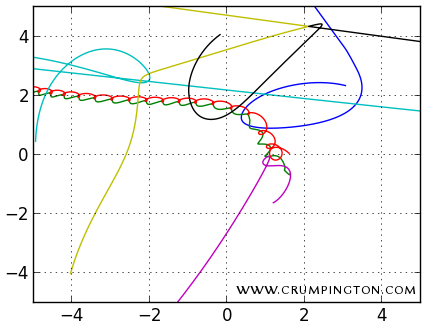您可以捐助，支持我们的公益事业。 1元 10元 50元 认证码：必填求知 文章 文库 Lib 视频 iPerson 课程 认证 咨询 工具 讲座 Modeler Code要资料订阅捐助1854 次浏览     评价： 好 中 差

 `# lib/sim.py class World(object): """World is a structure that holds the state of N bodies and additional variables. threads : (int) The number of threads to use for multithreaded implementations. STATE OF THE WORLD: N : (int) The number of bodies in the simulation. m : (1D ndarray) The mass of each body. r : (2D ndarray) The position of each body. v : (2D ndarray) The velocity of each body. F : (2D ndarray) The force on each body. TEMPORARY VARIABLES: Ft : (3D ndarray) A 2D force array for each thread's local storage. s : (2D ndarray) The vectors from one body to all others. s3 : (1D ndarray) The norm of each s vector. NOTE: Ft is used by parallel algorithms for thread-local storage. s and s3 are only used by the Python implementation. """ def __init__(self, N, threads=1, m_min=1, m_max=30.0, r_max=50.0, v_max=4.0, dt=1e-3): self.threads = threads self.N = N self.m = np.random.uniform(m_min, m_max, N) self.r = np.random.uniform(-r_max, r_max, (N, 2)) self.v = np.random.uniform(-v_max, v_max, (N, 2)) self.F = np.zeros_like(self.r) self.Ft = np.zeros((threads, N, 2)) self.s = np.zeros_like(self.r) self.s3 = np.zeros_like(self.m) self.dt = dt`

 `# lib/sim.py def compute_F(w): """Compute the force on each body in the world, w.""" for i in xrange(w.N): w.s[:] = w.r - w.r[i] w.s3[:] = (w.s[:,0]**2 + w.s[:,1]**2)**1.5 w.s3[i] = 1.0 # This makes the self-force zero. w.F[i] = (w.m[i] * w.m[:,None] * w.s / w.s3[:,None]).sum(0) def evolve(w, steps): """Evolve the world, w, through the given number of steps.""" for _ in xrange(steps): compute_F(w) w.v += w.F * w.dt / w.m[:,None] w.r += w.v * w.dt``import libw = lib.World(101)lib.evolve(w, 4096)`

 `python -m cProfile -scum bench.py`

 `428710 function calls (428521 primitive calls) in 16.836 secondsOrdered by: cumulative timencalls tottime percall cumtime percall filename:lineno(function) 1 0.000 0.000 16.837 16.837 bench.py:2() 1 0.062 0.062 16.756 16.756 sim.py:60(evolve) 4096 15.551 0.004 16.693 0.004 sim.py:51(compute_F)413696 1.142 0.000 1.142 0.000 {method 'sum' ... 3 0.002 0.001 0.115 0.038 __init__.py:1() ...`

 `static PyObject *evolve(PyObject *self, PyObject *args);`

 `static PyMethodDef methods[] = { { "evolve", evolve, METH_VARARGS, "Doc string."}, { NULL, NULL, 0, NULL } /* Sentinel */};`

 `PyMODINIT_FUNC initsimple1(void) { (void) Py_InitModule("simple1", methods); import_array();}`

 `#define m(x0) (*(npy_float64*)((PyArray_DATA(py_m) + \(x0) * PyArray_STRIDES(py_m))))#define m_shape(i) (py_m->dimensions[(i)]) #define r(x0, x1) (*(npy_float64*)((PyArray_DATA(py_r) + \(x0) * PyArray_STRIDES(py_r) + \(x1) * PyArray_STRIDES(py_r))))#define r_shape(i) (py_r->dimensions[(i)])`

 `for(i = 0; i < r_shape(0); ++i) { for(j = 0; j < r_shape(1); ++j) { r(i, j) = 0; // Zero all elements. }}`

 ```static inline void compute_F(npy_int64 N, PyArrayObject *py_m, PyArrayObject *py_r, PyArrayObject *py_F) { npy_int64 i, j; npy_float64 sx, sy, Fx, Fy, s3, tmp; // Set all forces to zero. for(i = 0; i < N; ++i) { F(i, 0) = F(i, 1) = 0; } // Compute forces between pairs of bodies. for(i = 0; i < N; ++i) { for(j = i + 1; j < N; ++j) { sx = r(j, 0) - r(i, 0); sy = r(j, 1) - r(i, 1); s3 = sqrt(sx*sx + sy*sy); s3 *= s3 * s3; tmp = m(i) * m(j) / s3; Fx = tmp * sx; Fy = tmp * sy; F(i, 0) += Fx; F(i, 1) += Fy; F(j, 0) -= Fx; F(j, 1) -= Fy; } } } ```

 ```static PyObject *evolve(PyObject *self, PyObject *args) { // Declare variables. npy_int64 N, threads, steps, step, i; npy_float64 dt; PyArrayObject *py_m, *py_r, *py_v, *py_F; // Parse arguments. if (!PyArg_ParseTuple(args, "ldllO!O!O!O!", &threads, &dt, &steps, &N, &PyArray_Type, &py_m, &PyArray_Type, &py_r, &PyArray_Type, &py_v, &PyArray_Type, &py_F)) { return NULL; } // Evolve the world. for(step = 0; step< steps; ++step) { compute_F(N, py_m, py_r, py_F); for(i = 0; i < N; ++i) { v(i, 0) += F(i, 0) * dt / m(i); v(i, 1) += F(i, 1) * dt / m(i); r(i, 0) += v(i, 0) * dt; r(i, 1) += v(i, 1) * dt; } } Py_RETURN_NONE; } ```

C版本的演化方法比Python版本更快，这应该不足为奇。在上面提到的相同的i5台式机中，C实现的演化方法能够实现每秒17972个时间步长。相比Python实现，这方面有70倍的提升。1854 次浏览     评价： 好 中 差订阅捐助
 相关文章 深度解析：清理烂代码 如何编写出拥抱变化的代码 重构-使代码更简洁优美 团队项目开发"编码规范"系列文章
 相关文档 重构-改善既有代码的设计 软件重构v2 代码整洁之道 高质量编程规范
 相关课程 基于HTML5客户端、Web端的应用开发 HTML 5+CSS 开发 嵌入式C高质量编程 C++高级编程讲座 前端开发框架uni-app 主讲：苏雯斐 时间：2020年8月15日实录 企业架构师的能力模型 主讲：俎涛

 WEB应用程序UI模版代码编写 C# 编码规范和编程好习惯 什么是防御性编程 善于防守-健壮代码的防御性 Visual C++编程命名规则 JavaScript程序编码规范

 设计模式原理与应用 从需求过渡到设计 软件设计原理与实践 如何编写高质量代码 单元测试、重构及持续集成 软件开发过程指南

 某全球知名通信公司 代码整洁 横河电机 如何编写高质量代码 某知名金融软件服务商 代码评审 东软集团 代码重构 某金融软件服务商 技术文档 中达电通 设计模式原理与实践 法国电信 技术文档编写与管理